# Legendre polynomials

(diff) ← Older revision | Latest revision (diff) | Newer revision → (diff)

spherical polynomials

Polynomials orthogonal on the interval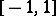with unit weight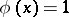. The standardized Legendre polynomials are defined by the Rodrigues formula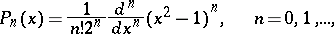and have the representation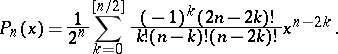The formulas most commonly used are: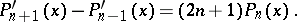The Legendre polynomials can be defined as the coefficients in the expansion of the generating functionwhere the series on the right-hand side converges for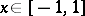.

The first few standardized Legendre polynomials have the form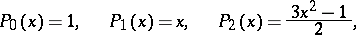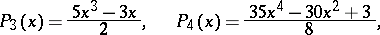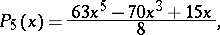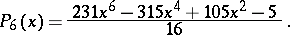The Legendre polynomial of ordersatisfies the differential equation (Legendre equation)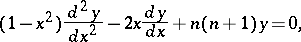which occurs in the solution of the Laplace equation in spherical coordinates by the method of separation of variables. The orthogonal Legendre polynomials have the form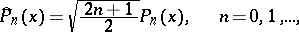and satisfy the uniform and weighted estimates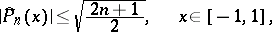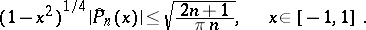Fourier series in the Legendre polynomials inside the intervalare analogous to trigonometric Fourier series (cf. also Fourier series in orthogonal polynomials); there is a theorem about the equiconvergence of these two series, which implies that the Fourier–Legendre series of a functionat a point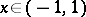converges if and only if the trigonometric Fourier series of the function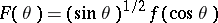converges at the point. In a neighbourhood of the end points the situation is different, since the sequence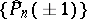increases with speed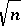. Ifis continuous onand satisfies a Lipschitz condition of order, then the Fourier–Legendre series converges touniformly on the whole interval. If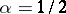, then this series generally diverges at the points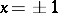.

These polynomials were introduced by A.M. Legendre .